# 【计算摄影学】双边滤波通俗理解以及简单实现

2020/03/13 15:55

## 概念

• 在图像的边缘部分，像素值的变化较为剧烈。
• 在图像的非边缘区域，像素值的变换较为平坦。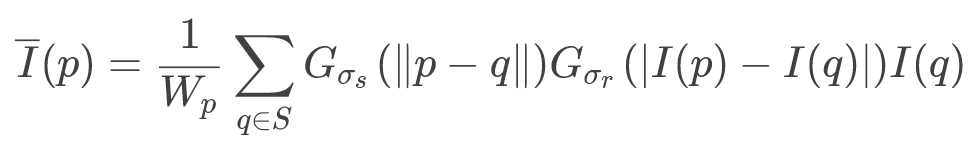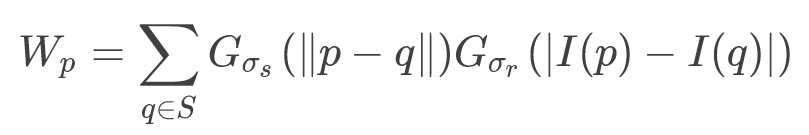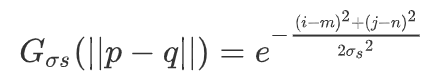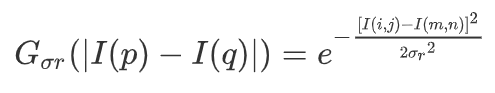在上面两个式子中，σs与σr都是已知的，或者说是自己输入的预设值，而其他的i，j，m，n都是需要我们在遍历中确定的值。其中（i，j）代表是窗口中心值，（m，n）代表的是滑动窗口中的某个值。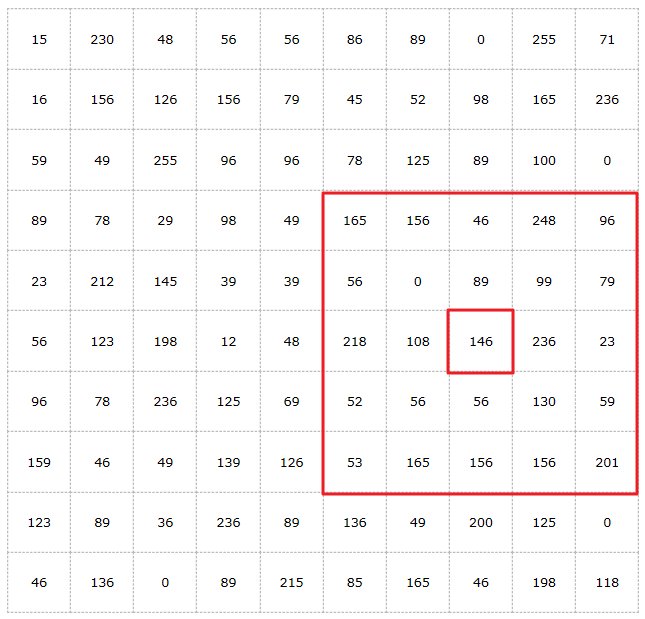整张图可以看作是10 × 10的一张图像，图中的数字表示每个点的像素值。在图中存在一个5 × 5大小的滑动窗口，我们需要求出中心点146的新像素值。

• 首先遍历整个窗口，第一个遍历到的点是165，那么中心点与该点的空间域计算结果为：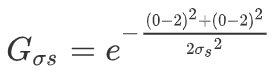• 再计算中心点与该点的像素域结果：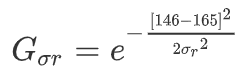• 当 σs 与 σr 分别为5和20时，Gσs = 0.8521，Gσr = 0.6368
• 接着遍历整个窗口，将窗口内每个像素点都与中心点建立联系，求出它们的 Gσs 与 Gσr 的值，将 Gσs 与 Gσr 相乘即得到每个点对应的Wp，即Wp = Gσs × Gσr
• 在遍历结束后，用每个点的Wp乘上该点的像素值I(m, n)，并求和，这是作为分子。将每个点的Wp相加，作为分母，两者相除，即得到需要的新输出图像的中心点（i，j）的像素值。

## 理论分析

• 读取原始图像。
• 设定窗口大小，遍历整个图像，将窗口覆盖内的所有像素值与窗口中心像素比对，求取空间域核与图像像素域核大小，并将两者相乘作为该点的特征值。
• 将每一点的特征值与该点像素值乘积相加，除以所有特征值的和，得到的结果即作为中心点的像素值。

## 实验细节

// 遍历图像
for (int i = 0; i < src.rows; i++) {
for (int j = 0; j < src.cols; j++) {
double fenzi_r, fenmu_r, fenzi_g, fenmu_g, fenzi_b, fenmu_b;
fenzi_r = fenmu_r = fenzi_g = fenmu_g = fenzi_b = fenmu_b = 0;

for (int m = i - window_size; m <= i + window_size; m++) {
for (int n = j - window_size; n <= j + window_size; n++) {
if (!(m<0 || m>src.rows - 1 || n<0 || n>src.cols - 1)) {
// 该条件排除了窗口内的图像边界外部值
double w = exp(-(1.0f)* (((m - i) * (m - i) + (n - j) * (n - j)) / (2.0f*sigma_s*sigma_s)));
double w_r = exp(-(1.0f)* (pow((double)(src.at<Vec3b>(i, j) - src.at<Vec3b>(m, n)), 2) / (2.0f*sigma_r*sigma_r)));
double w_g = exp(-(1.0f)* (pow((double)(src.at<Vec3b>(i, j) - src.at<Vec3b>(m, n)), 2) / (2.0f*sigma_r*sigma_r)));
double w_b = exp(-(1.0f)* (pow((double)(src.at<Vec3b>(i, j) - src.at<Vec3b>(m, n)), 2) / (2.0f*sigma_r*sigma_r)));
fenmu_r += w * w_r;
fenmu_g += w * w_g;
fenmu_b += w * w_b;
fenzi_r += w * w_r*(double)src.at<Vec3b>(m, n);
fenzi_g += w * w_g*(double)src.at<Vec3b>(m, n);
fenzi_b += w * w_b*(double)src.at<Vec3b>(m, n);
}
}
}

dst.at<Vec3b>(i, j) = (double)fenzi_r / (double)fenmu_r;
dst.at<Vec3b>(i, j) = (double)fenzi_g / (double)fenmu_g;
dst.at<Vec3b>(i, j) = (double)fenzi_b / (double)fenmu_b;
}
}

该部分为整个程序的主体部分。在该部分中，遍历图像的每一点，再在内部遍历以该点为中心点的窗口内部所有点。直接用公式求出每一点相对中心点的空间域的值，然后分为RGB三个通道求出像素域值。直接用分子、分母作为变量名，求出三个通道的所有需要的分子分母。在窗口循环结束后，直接作除法并赋值给目标输出图像即可。

## 成果展示（原始图像）（双边滤波处理图像，σs = 5，σr = 20）

0
0 收藏

### 作者的其它热门文章0 评论
0 收藏
0